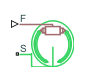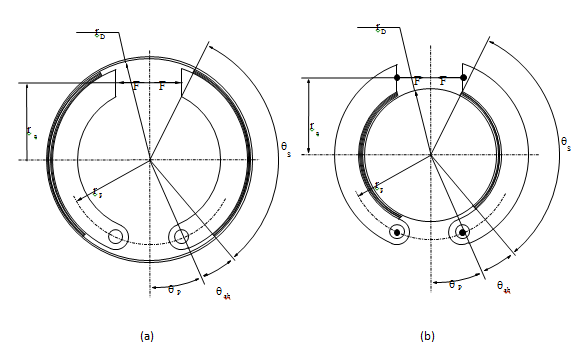# Double-Shoe Brake

Frictional brake with two pivoted shoes diametrically positioned about a rotating drum with triggered faulting

• Library:
• Simscape / Driveline / Brakes & Detents / Rotational

•## Description

The Double-Shoe Brake block represents a frictional brake with two pivoted rigid shoes that press against a rotating drum to produce a braking action. The rigid shoes sit inside or outside the rotating drum in a diametrically opposed configuration. A positive actuating force causes the rigid shoes to press against the rotating drum. Viscous and contact friction between the drum and the rigid shoe surfaces cause the rotating drum to decelerate.

Double-shoe brakes provide high braking torque with small actuator deflections in applications that include motor vehicles and some heavy machinery. The model employs a simple parameterization with readily accessible brake geometry and friction parameters.

You can also enable faulting. When faulting occurs, the belt will exert a user-specified force. Faults can occur at a specified time or due to an external trigger at port T.

### Equations

In the schematic, a) represents an internal double-shoe brake, and b) represents an external double-shoe brake. In both configurations, a positive actuation force F brings the shoe and drum friction surfaces into contact. The result is a friction torque that causes deceleration of the rotating drum. Zero and negative forces do not bring the shoe and drum friction surfaces into contact and produce zero braking torque.The model uses the long-shoe approximation. The equations for the friction torque that the leading and trailing shoes develop are:

`${T}_{LS}=\frac{c\mu {p}_{a}{r}_{D}{}^{2}\left(\mathrm{cos}{\theta }_{sb}-\mathrm{cos}{\theta }_{s}\right)}{\mathrm{sin}{\theta }_{a}},$`
`${T}_{TS}=\frac{c\mu {p}_{b}{r}_{D}{}^{2}\left(\mathrm{cos}{\theta }_{sb}-\mathrm{cos}{\theta }_{s}\right)}{\mathrm{sin}{\theta }_{a}},$`
`$c={r}_{a}+{r}_{p}\mathrm{cos}{\theta }_{p},$`

where for $0\le {\theta }_{s}\le \pi }{2}$,

`${\theta }_{a}={\theta }_{s},$`

and for ${\theta }_{s}\ge \pi }{2}$,

`${\theta }_{a}=\frac{\pi }{2}.$`

Where:

• TLS is the brake torque the leading shoe develops.

• TTS is the brake torque the trailing shoe develops.

• μ is the effective contact friction coefficient.

• pa is the maximum linear pressure in the leading shoe-drum contact.

• pb is the maximum linear pressure in the trailing shoe-drum contact.

• rD is the drum radius.

• θsb is the shoe beginning angle.

• θs is the shoe span angle.

• θa is the angle from hinge pin to maximum pressure point.

• c is the arm length of the cylinder force with respect to the hinge pin.

• rp is the pin location radius.

• θp is the hinge pin location angle.

• ra is the actuator location radius.

The model assumes that only Coulomb friction acts at the shoe-drum surface contact. Zero relative velocity between the drum and the shoes produces zero Coulomb friction. To avoid discontinuity at zero relative velocity, the friction coefficient formula employs the hyperbolic function

`$\mu ={\mu }_{Coulomb}\mathrm{tanh}\left(\frac{4{\omega }_{shaft}}{{\omega }_{threshold}}\right),$`

where:

• μ is the effective contact friction coefficient.

• μCoulomb is the contact friction coefficient.

• ωshaft is the shaft velocity.

• ωthreshold is the angular velocity threshold.

Balancing the moments that act on each shoe with respect to the pin yields the pressure acting at the shoe-drum surface contact. The equations for determining the balance of moments for the leading shoe are

`$F=\frac{{M}_{N}-{M}_{F}}{c},$`
`${M}_{N}=\frac{{p}_{a}{r}_{p}{r}_{D}}{\mathrm{sin}{\theta }_{a}}\left(\frac{1}{2}\left[{\theta }_{s}-{\theta }_{sb}\right]-\frac{1}{4}\left[\mathrm{sin}2{\theta }_{s}-\mathrm{sin}2{\theta }_{sb}\right]\right),$`

and

`${M}_{F}=\frac{\mu {p}_{a}{r}_{D}}{\mathrm{sin}{\theta }_{a}}\left({r}_{D}\left[\mathrm{cos}{\theta }_{sb}-\mathrm{cos}{\theta }_{s}\right]+\frac{{r}_{p}}{4}\left[\mathrm{cos}2{\theta }_{s}-\mathrm{cos}2{\theta }_{sb}\right]\right),$`

where:

• F is the actuation force.

• MN is the moment acting on the leading shoe due to normal force.

• MF is the moment acting on the leading shoe due to friction force.

• c is the arm length of the cylinder force with respect to the hinge pin.

• pa is the maximum linear pressure at the shoe-drum contact surface.

• rp is the pin location radius.

• θp is the hinge pin location angle.

• ra is the actuator location radius.

The model does not simulate self-locking brakes. If brake geometry and friction parameters cause a self-locking condition, the model produces a simulation error. A brake self-locks if the friction moment exceeds the moment due to normal forces, that is, when MF > MN.

The balance of moments for the trailing shoe is

`$F=\frac{{M}_{N}+{M}_{F}}{c}.$`

The net braking torque is

`$T={T}_{LS}+{T}_{TS}+{\mu }_{visc}*{\omega }_{shaft},$`

where μvisc is the viscous friction coefficient.

### Faults

When faults are enabled, a belt force is applied in response to one or both of these triggers:

• Simulation time — Faulting occurs at a specified time.

• Simulation behavior — Faulting occurs in response to an external trigger. This exposes port T.

If a fault trigger occurs, the input force is replaced by the Belt force when faulted value for the remainder of the simulation. A value of `0` implies that no braking will occur. A relatively large value implies that the brake is stuck.

You can set the block to issue a fault report as a warning or error message in the Simulink Diagnostic Viewer with the Reporting when fault occurs parameter.

### Thermal Model

You can model the effects of heat flow and temperature change by exposing the optional thermal port. To expose the port, in the Friction settings, set the Thermal Port parameter to `Model`. Exposing the port also exposes or changes the default value for these related settings, parameters, and variables:

• Friction > Temperature

• Friction > Static friction coefficient vector

• Friction > Coulomb friction coefficient vector

• Friction > Contact friction coefficient vector

• Thermal Port > Thermal mass

• Variables > Temperature

### Variables

Use the Variables settings to set the priority and initial target values for the block variables before simulating. For more information, see Set Priority and Initial Target for Block Variables.

Dependencies

Variable settings are visible only when, in the Friction settings, the Thermal port parameter is set to `Model`.

## Limitations and Assumptions

• Contact angles smaller than 45° produce less accurate results.

• The brake uses the long-shoe approximation.

• The brake geometry does not self-lock.

• The model does not account for actuator flow consumption.

## Ports

### Input

expand all

Physical signal input port associated with the applied actuating force.

Physical signal port for an external fault trigger. Triggering occurs when the value is greater than 0.5. There is no unit associated with the trigger value.

#### Dependencies

This port is visible when Enable faults is set to `On` and Enable external fault trigger is set to `On`.

### Conserving

expand all

Rotational conserving port associated with the rotating drum shaft.

Thermal conserving port associated with heat flow.

#### Dependencies

This port is visible only when, in the Friction settings, the Thermal Port parameter is set to `Model`.

Exposing this port makes related settings visible.

## Parameters

expand all

### Geometry

Radius of the drum contact surface. The value must be greater than zero.

Distance between the drum center and the force line of action. The value must be greater than zero.

Distance between the hinge pin and drum centers. The parameter must be greater than zero.

Angular coordinate of the hinge pin location from the brake symmetry axis. The value must be greater than or equal to zero.

Angle between the hinge pin and the beginning of the friction material linen of the shoe. The value of the parameter must be in the range0 ≤ θsb ≤ (π-pin location angle).

Angle between the beginning and the end of the friction material linen on the shoe. The value of the parameter must be in the range 0 < θsb ≤ (π -pin location angle - shoe beginning angle).

### Friction

Viscous friction coefficient at the contact surface. The value must be greater than or equal to zero.

Model for heat flow and temperature change:

• `Omit` — Neglect thermal dynamics.

• `Model` — Include thermal dynamics.

#### Dependencies

When this parameter is set to `Model`, thermal port H and related settings are visible.

Array of temperatures used to construct a 1-D temperature-efficiency lookup table. The array values must increase left to right.

#### Dependencies

This parameter is only visible when the Thermal Port parameter is set to `Model`.

Coulomb friction coefficient at the belt-drum contact surface. The value must be greater than zero. For the thermal model:

• The number of elements in the vector must be the same as the number of elements in the specified vector for the Temperature parameter

• The values increase left to right.

• Each value must be greater than zero.

#### Dependencies

This parameter is specified as a:

• Scalar when the Thermal Port parameter is set to `Omit`.

• Vector when the Thermal Port parameter is set to `Model`.

Angular velocity at which the contact friction coefficient practically reaches its steady-state value. The value must be greater than zero.

### Faults

Enable externally or temporally triggered faults. When faulting occurs, the brake belt force normally received at port F will be set to the value specified in the Belt force when faulted parameter.

Set faulted belt force. When faulting occurs, the brake belt force normally received at port F will be set to the value specified in the Belt force when faulted parameter. A value of `0` implies that braking does not occur. A relatively large value implies that the brake is stuck.

#### Dependencies

To enable this parameter, set Enable faults to `On`.

Enables port T. A physical signal at port T that is greater than `0.5` triggers faulting.

#### Dependencies

To enable this parameter, set Enable faults to `On`.

Enables fault triggering at a specified time. When the Simulation time for fault event is reached, the brake belt force normally received at port F will be set to the value specified in the Belt force when faulted parameter.

#### Dependencies

To enable this parameter, set Enable faults to `On`.

When the Simulation time for fault event is reached, the brake belt force normally received at port F will be set to the value specified in the Belt force when faulted parameter.

#### Dependencies

To enable this parameter, set Enable faults to `On` and Enable temporal fault trigger to `On`.

Reporting preference for the fault condition. When reporting is set to `Warning` or `Error`, a message is displayed in the Simulink Diagnostic Viewer. When `Error` is selected, the simulation will stop if faulting occurs.

#### Dependencies

To enable this parameter, set Enable faults to `On`.

### Thermal Port

Thermal energy required to change the component temperature by a single degree. The greater the thermal mass, the more resistant the component is to temperature change.

#### Dependencies

This parameter is only visible when, in the Friction settings, the Thermal Port parameter is set to `Model`.

## Version History

Introduced in R2012b